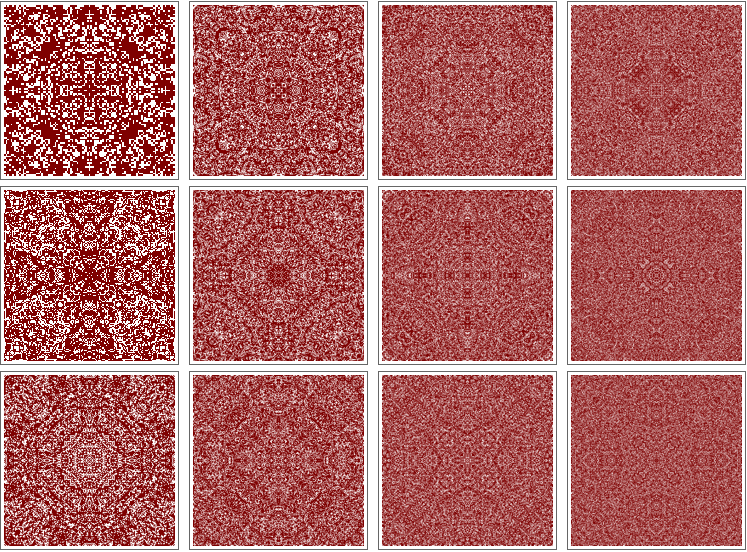#Wolfram Summer School

# Alumni# Réka Ágnes Kovács

Science and Technology

Class of 2016

## Bio

Réka has just finished her BSc in natural sciences at University College London, where she specialized in mathematics with a minor in neuroscience. This year she is starting her MSc in mathematical modeling and scientific computing at the University of Oxford.

Réka has been a passionate Mathematica programmer since her second year of undergraduate studies. She has one year of research experience from the Neural Computation Lab, Wolfson Institute for Biomedical Research at UCL, where she used Mathematica to develop a method that automates the detection of synaptic vesicles in electron microscopy images. This summer she will be working on a research project to investigate the cause of Zipf’s law in neural data at the Gatsby Computational Neuroscience Unit.

In her free time, she enjoys watching movies, listening to soundtracks of movies, reading about pure mathematics and visiting her hometown of Budapest.

## Project: Computational Analysis and Enumeration of Monoids and Pregroups

Lambek (1999) introduced a new computational method for deciding whether a sentence in natural language is grammatical. In his method, sentences are modeled as elements of mathematical structures called pregroups. A pregroup is a monoid with an extra structure that is based on a partial order over its elements and a set of rules for how pregroup element multiplication relates to the partial order. Lambek used infinite pregroups.

My project started with the enumeration of all possible multiplication tables for a set of size m=1,2,3,4. Then I developed functions to check how many of these multiplication tables correspond to a monoid. A monoid (S,·) is an algebraic structure consisting of a set S that is closed under a binary associative operation · and contains a unique identity element. In order to get the total number of non-isomorphic monoids for a certain size, I had to make sure that isomorphic monoids were not counted twice.

The next step was to add more axioms to the definition of monoids and arrive at the definition of pregroups. A pregroup is a quintuple (S, ·, ≤, l, r) consisting of a set S, a binary operation ·, a binary relation ≤ and two unary operations l, r. After my search for pregroups, it turned out that there aren’t any pregroups of size 1,2,3,4 that have nontrivial order. Based on this observation, we stated 4 lemmas that say that all finite pregroups are groups with trivial order.

After arriving at this conclusion, I decided to use protogroups to construct grammars. A protogroup is a quintuple
(S, ·, ≤, l, r), which satisfies less axioms than a pregroup but can also be used to construct grammars. I studied protogroup grammars that model the process of determining whether a sequence of words is grammatically correct or not.

Rule 11234323• 21st Annual Wolfram Summer School
• Bentley University, Waltham, MA, USA
• June 25–July 15, 2023